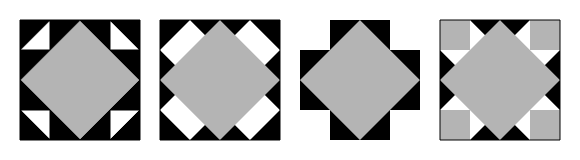## Exercises on Processing Programming

You should do all these exercises in Kotlin using the processing library. Problems marked SW are adaptation of exercises in Introduction to Programming In Java: An Interdisciplinary Approach, 2e, by Robert Sedgewick and Kevin Wayne.

1. Clock. Write a program that displays an animation of the hour, minute, and second of a digital clock. Use the processing frameRate() function to update the numbers roughly once per second.
2. Write a processing program that uses the sin() function to draw an image like this one.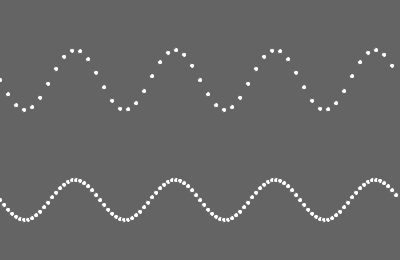3. First write a function pinwheel() that draws this image: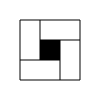Now write a program that takes a positive integer command-line argument $$n$$ and then draws an $$n$$-by-$$n$$ pinwheel mosaic. For example, $$n=5$$ gives this image: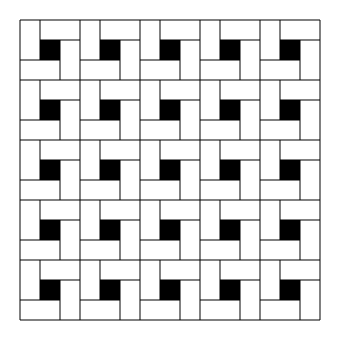4. (SW) Write a program that takes as command-line arguments an Int $$n$$ and a Float $$p$$ (between 0 and 1), plots $$n$$ equally spaced points on the circumference of a circle, and then, with probability $$p$$ for each pair of points, draws a gray line connecting them.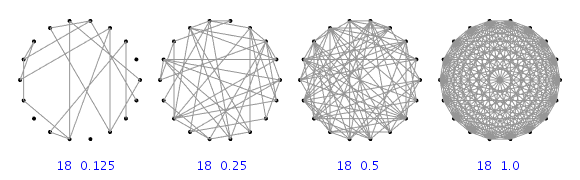5. (SW) Write functions to draw hearts, spades, clubs, and diamonds, one function per suit.
6. (SW) Write a program that takes an integer command-line argument $$n$$ and plots a rose with $$n$$ petals (if $$n$$ is odd) or $$2n$$ petals (if $$n$$ is even), by plotting the polar coordinates $$(r, \theta)$$ of the function $$r = \sin(n\theta)$$ for $$\theta$$ ranging from $$0$$ to $$2\pi$$ radians.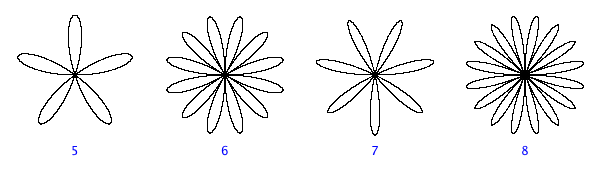7. Extending the previous exercise, write a program that takes a nonnegative integer command-line argument $$k$$ and plots a rose with $$k$$ petals. Here are the images you should get for $$k=1,2,3,4,5,6$$.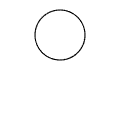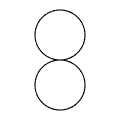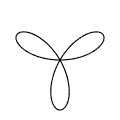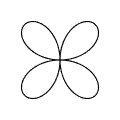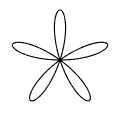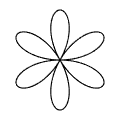What image should one get when $$k=0$$?
8. (SW) Write a program that takes a string command-line argument $$s$$ and displays it in banner style on the screen, moving from left to right and wrapping back to the beginning of the string as the end is reached.
9. (SW) Write programs that create each of the following designs.